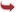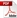International Journal of Scientific and Research Publications

#### IJSRP, Volume 2, Issue 12, December 2012 Edition [ISSN 2250-3153]Numerical Study on Heat Transfer of non-Newtonian Fluid Flow over Stretching Surface with Variable Viscosity in Uniform Magnetic Field
P. K. Mahanta
Abstract: An investigation has been carried out to obtain the flow and heat transfer of two dimensional electrically conducting second grade fluids over stretching surface in the presence of uniform magnetic field. The viscosity is assumed to vary as inverse linear function of temperature. The non-linear boundary layer equations together with the boundary conditions are reduced to a system of non-linear ordinary differential equations by using similarity transformations. The resulting equations are solved numerically by Runge-Kutta shooting method. The velocity and temperature profiles, the skin-friction and the rate of heat transfer are computed and discussed for different values of parameters. Some numerical results are presented graphically and discussed.
[VIEW FULL PAPER][DOWNLOAD]

Reference this Research Paper (copy & paste below code):

P. K. Mahanta (2018); Numerical Study on Heat Transfer of non-Newtonian Fluid Flow over Stretching Surface with Variable Viscosity in Uniform Magnetic Field; Int J Sci Res Publ 2(12) (ISSN: 2250-3153). http://www.ijsrp.org/research-paper-1212.php?rp=P12478